##### Economics of Money: Chapter 5

+3
Set Details Share
created 3 years ago by powerup
20,176 views
The Behavior of Interest Rates
Page to share:
Embed this setcancel
COPY
code changes based on your size selection
Size:
X
1

Pieces of property that serve as a store of value are called

1. A) assets.
2. B) units of account.
3. C) liabilities.
4. D) borrowings.

2

Of the four factors that influence asset demand, which factor will cause the demand for all assets to increase when it increases, everything else held constant?

1. A) wealth
2. B) expected returns
3. C) risk
4. D) liquidity

3

If wealth increases, the demand for stocks ________ and that of long-term bonds ________, everything else held constant.

1. A) increases; increases
2. B) increases; decreases
3. C) decreases; decreases
4. D) decreases; increases

4

Everything else held constant, a decrease in wealth

1. A) increases the demand for stocks.
2. B) increases the demand for bonds.
3. C) reduces the demand for silver.
4. D) increases the demand for gold.

5

An increase in an asset's expected return relative to that of an alternative asset, holding everything else constant, ________ the quantity demanded of the asset.

1. A) increases
2. B) decreases
3. C) has no effect on
4. D) erases

6

Everything else held constant, if the expected return on ABC stock rises from 5 to 10 percent and the expected return on CBS stock is unchanged, then the expected return of holding CBS stock ________ relative to ABC stock and the demand for CBS stock ________.

1. A) rises; rises
2. B) rises; falls
3. C) falls; rises
4. D) falls; falls

7

Everything else held constant, if the expected return on U.S. Treasury bonds falls from 10 to 5 percent and the expected return on GE stock rises from 7 to 8 percent, then the expected return of holding GE stock ________ relative to U.S. Treasury bonds and the demand for GE stock ________.

1. A) rises; rises
2. B) rises; falls
3. C) falls; rises
4. D) falls; falls

8

If housing prices are expected to increase, then, other things equal, the demand for houses will ________ and that of Treasury bills will ________.

1. A) increase; increase
2. B) increase; decrease
3. C) decrease; decrease
4. D) decrease; increase

9

If stock prices are expected to drop dramatically, then, other things equal, the demand for stocks will ________ and that of Treasury bills will ________.

1. A) increase; increase
2. B) increase; decrease
3. C) decrease; decrease
4. D) decrease; increase

10

Everything else held constant, if the expected return on RST stock declines from 12 to 9 percent and the expected return on XYZ stock declines from 8 to 7 percent, then the expected return of holding RST stock ________ relative to XYZ stock and demand for XYZ stock ________.

1. A) rises; rises
2. B) rises; falls
3. C) falls; rises
4. D) falls; falls

11

Everything else held constant, if the expected return on U.S. Treasury bonds falls from 8 to 7 percent and the expected return on corporate bonds falls from 10 to 8 percent, then the expected return of corporate bonds ________ relative to U.S. Treasury bonds and the demand for corporate bonds ________.

1. A) rises; rises
2. B) rises; falls
3. C) falls; rises
4. D) falls; falls

12

An increase in the expected rate of inflation will ________ the expected return on bonds relative to the that on ________ assets, everything else held constant.

1. A) reduce; financial
2. B) reduce; real
3. C) raise; financial
4. D) raise; real

13

If fluctuations in interest rates become smaller, then, other things equal, the demand for stocks ________ and the demand for long-term bonds ________.

1. A) increases; increases
2. B) increases; decreases
3. C) decreases; decreases
4. D) decreases; increases

14

If the price of gold becomes less volatile, then, other things equal, the demand for stocks will ________ and the demand for antiques will ________.

1. A) increase; increase
2. B) increase; decrease
3. C) decrease; decrease
4. D) decrease; increase

15

If brokerage commissions on bond sales decrease, then, other things equal, the demand for bonds will ________ and the demand for real estate will ________.

1. A) increase; increase
2. B) increase; decrease
3. C) decrease; decrease
4. D) decrease; increase

16

If gold becomes acceptable as a medium of exchange, the demand for gold will ________ and the demand for bonds will ________, everything else held constant.

1. A) decrease; decrease
2. B) decrease; increase
3. C) increase; increase
4. D) increase; decrease

17

The demand for Picasso paintings rises (holding everything else equal) when

1. A) stocks become easier to sell.
2. B) people expect a boom in real estate prices.
3. C) Treasury securities become riskier.
4. D) people expect gold prices to rise.

18

The demand for silver decreases, other things equal, when

1. A) the gold market is expected to boom.
2. B) the market for silver becomes more liquid.
3. C) wealth grows rapidly.
4. D) interest rates are expected to rise.

19

You would be less willing to purchase U.S. Treasury bonds, other things equal, if

1. A) you inherit \$1 million from your Uncle Harry.
2. B) you expect interest rates to fall.
3. C) gold becomes more liquid.
4. D) stock prices are expected to fall.

20

You would be more willing to buy AT&T bonds (holding everything else constant) if

1. A) the brokerage commissions on bond sales become cheaper.
2. B) interest rates are expected to rise.
3. C) your wealth has decreased.
4. D) you expect diamonds to appreciate in value.

21

The demand for gold increases, other things equal, when

1. A) the market for silver becomes more liquid.
2. B) interest rates are expected to rise.
3. C) interest rates are expected to fall.
4. D) real estate prices are expected to increase.

22

The demand for houses decreases, all else equal, when

1. A) wealth increases.
2. B) real estate prices are expected to increase.
3. C) stock prices become more volatile.
4. D) gold prices are expected to increase.

23

Holding everything else constant

1. A) if asset A's risk rises relative to that of alternative assets, the demand will increase for asset A.
2. B) the more liquid is asset A, relative to alternative assets, the greater will be the demand for asset A.
3. C) the lower the expected return to asset A relative to alternative assets, the greater will be the demand for asset A.
4. D) if wealth increases, demand for asset A increases and demand for alternative assets decreases.

24

Holding all other factors constant, the quantity demanded of an asset is

1. A) positively related to wealth.
2. B) negatively related to its expected return relative to alternative assets.
3. C) positively related to the risk of its returns relative to alternative assets.
4. D) negatively related to its liquidity relative to alternative assets.

25

If the price of diamonds is expected to decrease, all else equal, then the demand for diamonds ________ and the demand for platinum ________.

1. A) decreases; increases
2. B) decreases; decreases
3. C) increases; increases
4. D) increases; decreases

26

If prices in the diamond market become less volatile, all else equal, then the demand for diamonds ________ and the demand for gold ________.

1. A) increases; decreases
2. B) increases; increases
3. C) decreases; decreases
4. D) decreases; increases

27

Everything else held constant, would an increase in volatility of stock prices have any impact on the demand for rare coins? Why or why not?

Answer: Yes, it would cause the demand for rare coins to increase. The increased volatility of stock prices means that there is relatively more risk in owning stock than there was previously and so the demand for an alternative asset, rare coins, would increase.

28

In the bond market, the bond demanders are the ________ and the bond suppliers are the ________.

1. A) lenders; borrowers
2. B) lenders; advancers
3. C) borrowers; lenders
4. D) borrowers; advancers

29

The demand curve for bonds has the usual downward slope, indicating that at ________ prices of the bond, everything else equal, the ________ is higher.

1. A) higher; demand
2. B) higher; quantity demanded
3. C) lower; demand
4. D) lower; quantity demanded

30

The bond demand curve is ________ sloping, indicating a(n) ________ relationship between the price and quantity demanded of bonds, everything else equal.

1. A) downward; inverse
2. B) downward; direct
3. C) upward; inverse
4. D) upward; direct

31

The supply curve for bonds has the usual upward slope, indicating that as the price ________, ceteris paribus, the ________ increases.

1. A) falls; supply
2. B) falls; quantity supplied
3. C) rises; supply
4. D) rises; quantity supplied

32

The bond supply curve is ________ sloping, indicating a(n) ________ relationship between the price and quantity supplied of bonds, everything else equal.

1. A) downward; inverse
2. B) downward; direct
3. C) upward; inverse
4. D) upward; direct

33

In the bond market, the market equilibrium shows the market-clearing ________ and market-clearing ________.

1. A) price; deposit
2. B) interest rate; deposit
3. C) price; interest rate
4. D) interest rate; premium

34

When the price of a bond is above the equilibrium price, there is an excess ________ bonds and price will ________.

1. A) demand for; rise
2. B) demand for; fall
3. C) supply of; fall
4. D) supply of; rise

35

When the price of a bond is ________ the equilibrium price, there is an excess demand for bonds and price will ________.

1. A) above; rise
2. B) above; fall
3. C) below; fall
4. D) below; rise

36

When the interest rate on a bond is above the equilibrium interest rate, in the bond market there is excess ________ and the interest rate will ________.

1. A) demand; rise
2. B) demand; fall
3. C) supply; fall
4. D) supply; rise

37

When the interest rate on a bond is ________ the equilibrium interest rate, in the bond market there is excess ________ and the interest rate will ________.

1. A) above; demand; rise
2. B) above; demand; fall
3. C) below; supply; fall
4. D) above; supply; rise

38

A situation in which the quantity of bonds supplied exceeds the quantity of bonds demanded is called a condition of excess supply; because people want to sell ________ bonds than others want to buy, the price of bonds will ________.

1. A) fewer; fall
2. B) fewer; rise
3. C) more; fall
4. D) more; rise

39

If the price of bonds is set ________ the equilibrium price, the quantity of bonds demanded exceeds the quantity of bonds supplied, a condition called excess ________.

1. A) above; demand
2. B) above; supply
3. C) below; demand
4. D) below; supply

40

If the interest rate on a bond is above the equilibrium interest rate, there is an excess ________ for bonds and the bond price will ________.

1. A) demand; rise
2. B) demand; fall
3. C) supply; rise
4. D) supply; fall

41

If the interest rate on a bond is below the equilibrium interest rate, there is an excess ________ of bonds and the bond price will ________.

1. A) demand; rise
2. B) demand; fall
3. C) supply; rise
4. D) supply; fall

42

A movement along the bond demand or supply curve occurs when ________ changes.

1. A) bond price
2. B) income
3. C) wealth
4. D) expected return

43

When the price of a bond decreases, all else equal, the bond demand curve

1. A) shifts right.
2. B) shifts left.
3. C) does not shift.
4. D) inverts.

44

During business cycle expansions when income and wealth are rising, the demand for bonds ________ and the demand curve shifts to the ________, everything else held constant.

1. A) falls; right
2. B) falls; left
3. C) rises; right
4. D) rises; left

45

Everything else held constant, when households save less, wealth and the demand for bonds ________ and the bond demand curve shifts ________.

1. A) increase; right
2. B) increase; left
3. C) decrease; right
4. D) decrease; left

46

Everything else held constant, if interest rates are expected to fall in the future, the demand for long-term bonds today ________ and the demand curve shifts to the ________.

1. A) rises; right
2. B) rises; left
3. C) falls; right
4. D) falls; left

47

Holding everything else constant, if interest rates are expected to increase, the demand for bonds ________ and the demand curve shifts ________.

1. A) increases; right
2. B) decreases; right
3. C) increases; left
4. D) decreases; left

48

Holding the expected return on bonds constant, an increase in the expected return on common stocks would ________ the demand for bonds, shifting the demand curve to the ________.

1. A) decrease; left
2. B) decrease; right
3. C) increase; left
4. D) increase; right

49

Everything else held constant, an increase in expected inflation, lowers the expected return on ________ compared to ________ assets.

1. A) bonds; financial
2. B) bonds; real
3. C) real estate; financial
4. D) real estate; real

50

Everything else held constant, an increase in the riskiness of bonds relative to alternative assets causes the demand for bonds to ________ and the demand curve to shift to the ________.

1. A) rise; right
2. B) rise; left
3. C) fall; right
4. D) fall; left

51

Everything else held constant, when stock prices become less volatile, the demand curve for bonds shifts to the ________ and the interest rate ________.

1. A) right; rises
2. B) right; falls
3. C) left; falls
4. D) left; rises

52

Everything else held constant, when stock prices become ________ volatile, the demand curve for bonds shifts to the ________ and the interest rate ________.

1. A) more; right; rises
2. B) more; right; falls
3. C) less; left; falls
4. D) less; left; does not change

53

Everything else held constant, an increase in the liquidity of bonds results in a ________ in demand for bonds and the demand curve shifts to the ________.

1. A) rise; right
2. B) rise; left
3. C) fall; right
4. D) fall; left

54

Everything else held constant, when bonds become less widely traded, and as a consequence the market becomes less liquid, the demand curve for bonds shifts to the ________ and the interest rate ________.

1. A) right; rises
2. B) right; falls
3. C) left; falls
4. D) left; rises

55

The reduction of brokerage commissions for trading common stocks that occurred in 1975 caused the demand for bonds to ________ and the demand curve to shift to the ________.

1. A) fall; right
2. B) fall, left
3. C) rise; right
4. D) rise; left

56

Factors that decrease the demand for bonds include

1. A) an increase in the volatility of stock prices.
2. B) a decrease in the expected returns on stocks.
3. C) a decrease in the inflation rate.
4. D) a decrease in the riskiness of stocks.

57

During a recession, the supply of bonds ________ and the supply curve shifts to the ________, everything else held constant.

1. A) increases; left
2. B) increases; right
3. C) decreases; left
4. D) decreases; right

58

In a business cycle expansion, the ________ of bonds increases and the ________ curve shifts to the ________ as business investments are expected to be more profitable.

1. A) supply; supply; right
2. B) supply; supply; left
3. C) demand; demand; right
4. D) demand; demand; left

59

When the expected inflation rate increases, the real cost of borrowing ________ and bond supply ________, everything else held constant.

1. A) increases; increases
2. B) increases; decreases
3. C) decreases; increases
4. D) decreases; decreases

60

An increase in the expected inflation rate causes the supply of bonds to ________ and the supply curve to shift to the ________, everything else held constant.

1. A) increase; left
2. B) increase; right
3. C) decrease; left
4. D) decrease; right

61

Higher government deficits ________ the supply of bonds and shift the supply curve to the ________, everything else held constant.

1. A) increase; left
2. B) increase; right
3. C) decrease; left
4. D) decrease; right

62

Factors that can cause the supply curve for bonds to shift to the right include

1. A) an expansion in overall economic activity.
2. B) a decrease in expected inflation.
3. C) a decrease in government deficits.
4. D) a business cycle recession.

63

When the inflation rate is expected to increase, the ________ for bonds falls, while the ________ curve shifts to the right, everything else held constant.

1. A) demand; demand
2. B) demand; supply
3. C) supply; demand
4. D) supply; supply

64

When the expected inflation rate increases, the demand for bonds ________, the supply of bonds ________, and the interest rate ________, everything else held constant.

1. A) increases; increases; rises
2. B) decreases; decreases; falls
3. C) increases; decreases; falls
4. D) decreases; increases; rises

65

Everything else held constant, when the inflation rate is expected to rise, interest rates will ________; this result has been termed the ________.

1. A) fall; Keynes effect
2. B) fall; Fisher effect
3. C) rise; Keynes effect
4. D) rise; Fisher effect

66

The economist Irving Fisher, after whom the Fisher effect is named, explained why interest rates ________ as the expected rate of inflation ________, everything else held constant.

1. A) rise; increases
2. B) rise; stabilizes
3. C) fall; stabilizes
4. D) fall; increases

67

Everything else held constant, during a business cycle expansion, the supply of bonds shifts to the ________ as businesses perceive more profitable investment opportunities, while the demand for bonds shifts to the ________ as a result of the increase in wealth generated by the economic expansion.

1. A) right; left
2. B) right; right
3. C) left; left
4. D) left; right

68

When the economy slips into a recession, normally the demand for bonds ________, the supply of bonds ________, and the interest rate ________, everything else held constant.

1. A) increases; increases; rises
2. B) decreases; decreases; falls
3. C) increases; decreases; falls
4. D) decreases; increases; rises

69

When an economy grows out of a recession, normally the demand for bonds ________ and the supply of bonds ________, everything else held constant.

1. A) increases; increases
2. B) increases; decreases
3. C) decreases; decreases
4. D) decreases; increases

70

Deflation causes the demand for bonds to ________, the supply of bonds to ________, and bond prices to ________, everything else held constant.

1. A) increase; increase; increase
2. B) increase; decrease; increase
3. C) decrease; increase; increase
4. D) decrease; decrease; increase

71

In the 1990s Japan had the lowest interest rates in the world due to a combination of

1. A) inflation and recession.
2. B) deflation and expansion.
3. C) inflation and expansion.
4. D) deflation and recession.

72

When the interest rate changes,

1. A) the demand curve for bonds shifts to the right.
2. B) the demand curve for bonds shifts to the left.
3. C) the supply curve for bonds shifts to the right.
4. D) it is because either the demand or the supply curve has shifted.

73

The interest rate falls when either the demand for bonds ________ or the supply of bonds ________.

1. A) increases; increases
2. B) increases; decreases
3. C) decreases; decreases
4. D) decreases; increases

74

When the government has a surplus, as occurred in the late 1990s, the ________ curve of bonds shifts to the ________, everything else held constant.

1. A) supply; right
2. B) supply; left
3. C) demand; right
4. D) demand; left

75

A decrease in the brokerage commissions in the housing market from 6% to 5% of the sales price will shift the ________ curve for bonds to the ________, everything else held constant.

1. A) demand; right
2. B) demand; left
3. C) supply; right
4. D) supply; left

76

When the prices of rare coins become volatile, the ________ curve for bonds shifts to the ________, everything else held constant.

1. A) demand; right
2. B) demand; left
3. C) supply; right
4. D) supply; left

77

If people expect real estate prices to increase significantly, the ________ curve for bonds will shift to the ________, everything else held constant.

1. A) demand; right
2. B) demand; left
3. C) supply; left
4. D) supply; right

78

Everything else held constant, when prices in the art market become more uncertain

1. A) the demand curve for bonds shifts to the left and the interest rate rises.
2. B) the demand curve for bonds shifts to the left and the interest rate falls.
3. C) the demand curve for bonds shifts to the right and the interest rate falls.
4. D) the supply curve for bonds shifts to the right and the interest rate falls.

79

Everything else held constant, when real estate prices are expected to decrease

1. A) the demand curve for bonds shifts to the left and the interest rate rises.
2. B) the demand curve for bonds shifts to the left and the interest rate falls.
3. C) the demand curve for bonds shifts to the right and the interest rate falls.
4. D) the supply curve for bonds shifts to the right and the interest rate falls.

80

Everything else held constant, when the government has higher budget deficits

1. A) the demand curve for bonds shifts to the left and the interest rate rises.
2. B) the demand curve for bonds shifts to the left and the interest rate falls.
3. C) the supply curve for bonds shifts to the right and the interest rate falls.
4. D) the supply curve for bonds shifts to the right and the interest rate rises.

81

If stock prices are expected to climb next year, everything else held constant, the ________ curve for bonds shifts ________ and the interest rate ________.

1. A) demand; left; rises
2. B) demand; right; rises
3. C) demand; left; falls
4. D) supply; left; rises

82

If prices in the bond market become more volatile, everything else held constant, the demand curve for bonds shifts ________ and interest rates ________.

1. A) left; rise
2. B) left; fall
3. C) right; rise
4. D) right; fall

83

If brokerage commissions on stocks fall, everything else held constant, the demand for bonds ________, the price of bonds ________, and the interest rate ________.

1. A) decreases; decreases; increases
2. B) decreases; decreases; decreases
3. C) increases; decreases; increases
4. D) increases; increases; increases

84

If the expected return on bonds increases, all else equal, the demand for bonds increases, the price of bonds ________, and the interest rate ________.

1. A) increases; decreases
2. B) increases; increases
3. C) decreases; decreases
4. D) decreases; increases

85

If real estate prices are expected to drop, all else equal, the demand for bonds ________ and the interest rate_______.

1. A) increases; rises
2. B) increases; falls
3. C) decreases; rises
4. D) decreases; falls

86In the figure above, a factor that could cause the supply of bonds to shift to the right is

1. A) a decrease in government budget deficits.
2. B) a decrease in expected inflation.
3. C) a recession.
4. D) a business cycle expansion.

87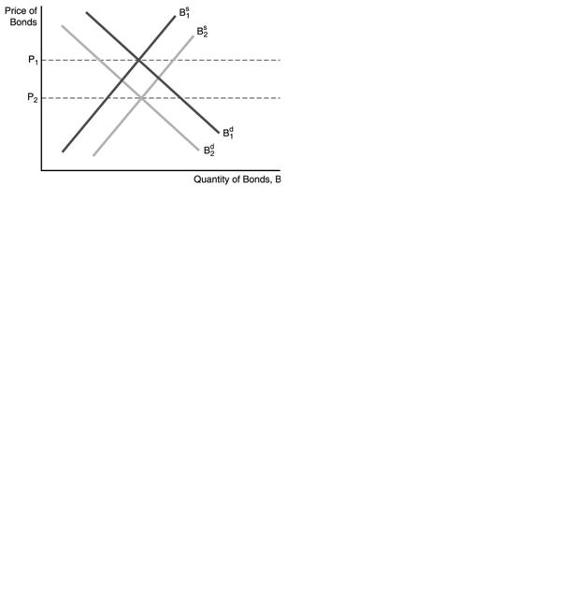In the figure above, a factor that could cause the demand for bonds to decrease (shift to the left) is

1. A) an increase in the expected return on bonds relative to other assets.
2. B) a decrease in the expected return on bonds relative to other assets.
3. C) an increase in wealth.
4. D) a reduction in the riskiness of bonds relative to other assets.

88In the figure above, the price of bonds would fall from P1 to P2 when

1. A) inflation is expected to increase in the future.
2. B) interest rates are expected to fall in the future.
3. C) the expected return on bonds relative to other assets is expected to increase in the future.
4. D) the riskiness of bonds falls relative to other assets.

89In the figure above, a factor that could cause the supply of bonds to increase (shift to the right) is

1. A) a decrease in government budget deficits.
2. B) a decrease in expected inflation.
3. C) expectations of more profitable investment opportunities.
4. D) a business cycle recession.

90In the figure above, a factor that could cause the demand for bonds to shift to the right is

1. A) an increase in the riskiness of bonds relative to other assets.
2. B) an increase in the expected rate of inflation.
3. C) expectations of lower interest rates in the future.
4. D) a decrease in wealth.

91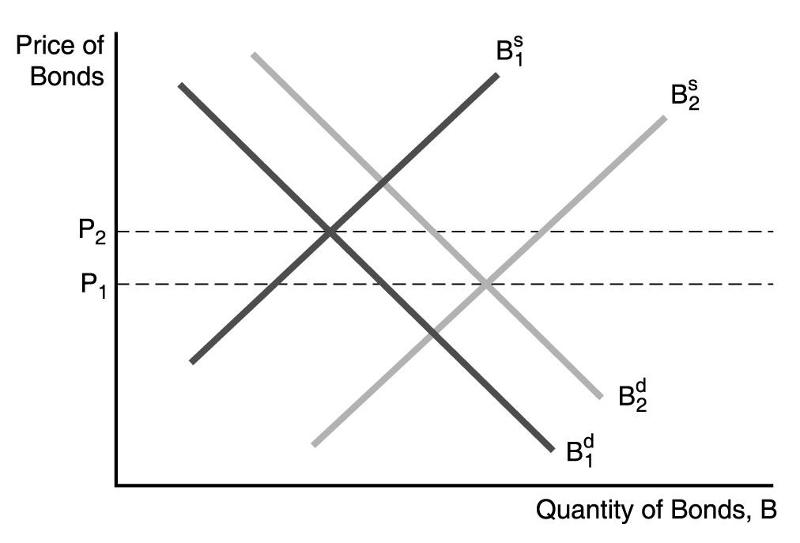In the figure above, the price of bonds would fall from P2 to P1 if

1. A) there is a business cycle recession.
2. B) there is a business cycle expansion.
3. C) inflation is expected to increase in the future.
4. D) inflation is expected to decrease in the future.

92

What is the impact on interest rates when the Federal Reserve decreases the money supply by selling bonds to the public?

Answer: Bond supply increases and the bond supply curve shifts to the right. The new equilibrium bond price is lower and thus interest rates will increase.

93

Use demand and supply analysis to explain why an expectation of Fed rate hikes would cause Treasury prices to fall.

Answer: The expected return on bonds would decrease relative to other assets resulting in a decrease in the demand for bonds. The leftward shift of the bond demand curve results in a new lower equilibrium price for bonds.

94

In Keynes's liquidity preference framework, individuals are assumed to hold their wealth in two forms

1. A) real assets and financial assets.
2. B) stocks and bonds.
3. C) money and bonds.
4. D) money and gold.

95

In Keynes's liquidity preference framework

1. A) the demand for bonds must equal the supply of money.
2. B) the demand for money must equal the supply of bonds.
3. C) an excess demand of bonds implies an excess demand for money.
4. D) an excess supply of bonds implies an excess demand for money.

96

In Keynes's liquidity preference framework, if there is excess demand for money, there is

1. A) an excess demand for bonds.
2. B) equilibrium in the bond market.
3. C) an excess supply of bonds.
4. D) too much money.

97

The bond supply and demand framework is easier to use when analyzing the effects of changes in ________, while the liquidity preference framework provides a simpler analysis of the effects from changes in income, the price level, and the supply of ________.

1. A) expected inflation; bonds
2. B) expected inflation; money
3. C) government budget deficits; bonds
4. D) government budget deficits; money

98

Keynes assumed that money has ________ rate of return.

1. A) a positive
2. B) a negative
3. C) a zero
4. D) an increasing

99

In his Liquidity Preference Framework, Keynes assumed that money has a zero rate of return; thus

1. A) when interest rates rise, the expected return on money falls relative to the expected return on bonds, causing the demand for money to fall.
2. B) when interest rates rise, the expected return on money falls relative to the expected return on bonds, causing the demand for money to rise.
3. C) when interest rates fall, the expected return on money falls relative to the expected return on bonds, causing the demand for money to fall.
4. D) when interest rates fall, the expected return on money falls relative to the expected return on bonds, causing the demand for money to rise.

100

In Keynes's liquidity preference framework, as the expected return on bonds increases (holding everything else unchanged), the expected return on money ________, causing the demand for ________ to fall.

1. A) falls; bonds
2. B) falls; money
3. C) rises; bonds
4. D) rises; money

101

The opportunity cost of holding money is

1. A) the level of income.
2. B) the price level.
3. C) the interest rate.
4. D) the discount rate.

102

An increase in the interest rate

1. A) increases the demand for money.
2. B) increases the quantity of money demanded.
3. C) decreases the demand for money.
4. D) decreases the quantity of money demanded.

103

If there is an excess supply of money

1. A) individuals sell bonds, causing the interest rate to rise.
2. B) individuals sell bonds, causing the interest rate to fall.
3. C) individuals buy bonds, causing interest rates to fall.
4. D) individuals buy bonds, causing interest rates to rise.

104

If there is an excess demand for money, individuals ________ bonds, causing interest rates to ________.

1. A) sell; rise
2. B) sell; fall
3. C) buy; rise
4. D) buy; fall

105

When the interest rate is above the equilibrium interest rate, there is an excess ________ money and the interest rate will ________.

1. A) demand for; rise
2. B) demand for; fall
3. C) supply of; fall
4. D) supply of; rise

106

In the market for money, an interest rate below equilibrium results in an excess ________ money and the interest rate will ________.

1. A) demand for; rise
2. B) demand for; fall
3. C) supply of; fall
4. D) supply of; rise

107

In the Keynesian liquidity preference framework, an increase in the interest rate causes the demand curve for money to ________, everything else held constant.

1. A) shift right
2. B) shift left
3. C) stay where it is
4. D) invert

108

A lower level of income causes the demand for money to ________ and the interest rate to ________, everything else held constant.

1. A) decrease; decrease
2. B) decrease; increase
3. C) increase; decrease
4. D) increase; increase

109

When real income ________, the demand curve for money shifts to the ________ and the interest rate ________, everything else held constant.

1. A) falls; right; rises
2. B) rises; right; rises
3. C) falls; left; rises
4. D) rises; left; rises

110

A business cycle expansion increases income, causing money demand to ________ and interest rates to ________, everything else held constant.

1. A) increase; increase
2. B) increase; decrease
3. C) decrease; decrease
4. D) decrease; increase

111

In the Keynesian liquidity preference framework, a rise in the price level causes the demand for money to ________ and the demand curve to shift to the ________, everything else held constant.

1. A) increase; left
2. B) increase; right
3. C) decrease; left
4. D) decrease; right

112

When the price level ________, the demand curve for money shifts to the ________ and the interest rate ________, everything else held constant.

1. A) falls; right; rises
2. B) rises; right; falls
3. C) falls; left; rises
4. D) rises; right; rises

113

A rise in the price level causes the demand for money to ________ and the interest rate to ________, everything else held constant.

1. A) decrease; decrease
2. B) decrease; increase
3. C) increase; decrease
4. D) increase; increase

114

When the price level falls, the ________ curve for nominal money ________, and interest rates ________, everything else held constant.

1. A) demand; decreases; fall
2. B) demand; increases; rise
3. C) supply; increases; rise
4. D) supply; decreases; fall

115

A decline in the expected inflation rate causes the demand for money to ________ and the demand curve to shift to the ________, everything else held constant.

1. A) decrease; right
2. B) decrease; left
3. C) increase; right
4. D) increase; left

116

When the Fed decreases the money stock, the money supply curve shifts to the ________ and the interest rate ________, everything else held constant.

1. A) right; rises
2. B) right; falls
3. C) left; falls
4. D) left; rises

117

When the Fed ________ the money stock, the money supply curve shifts to the ________ and the interest rate ________, everything else held constant.

1. A) decreases; right; rises
2. B) increases; right; falls
3. C) decreases; left; falls
4. D) increases; left; rises

118

________ in the money supply creates excess ________ money, causing interest rates to ________, everything else held constant.

1. A) A decrease; demand for; rise
2. B) An increase; demand for; fall
3. C) An increase; supply of; rise
4. D) A decrease; supply of; fall

119

________ in the money supply creates excess demand for ________, causing interest rates to ________, everything else held constant.

1. A) An increase; money; rise
2. B) An increase; bonds; fall
3. C) A decrease; bonds; rise
4. D) A decrease; money; fall

120

When the price level falls, the ________ curve for nominal money ________, and interest rates ________, everything else held constant.

1. A) demand; decreases; fall
2. B) demand; increases; rise
3. C) supply; increases; rise
4. D) supply; decreases; fall

121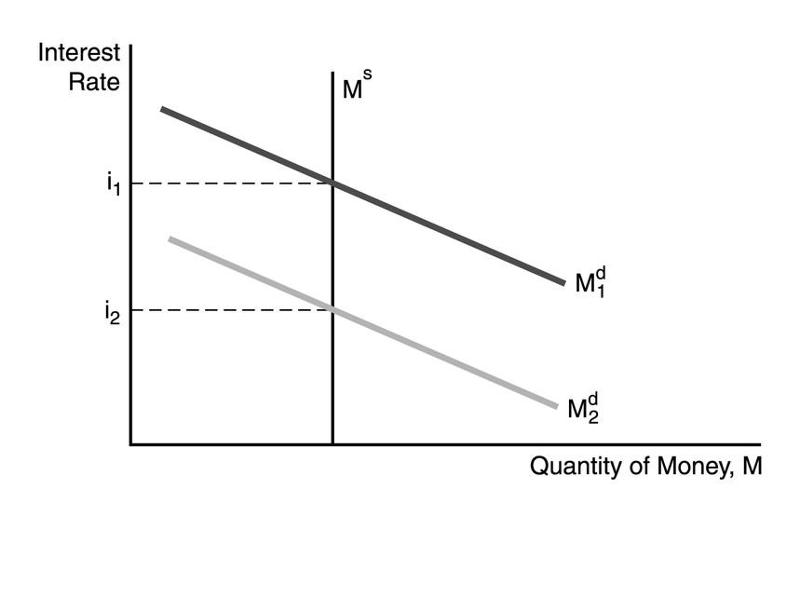In the figure above, one factor NOT responsible for the decline in the demand for money is

1. A) a decline the price level.
2. B) a decline in income.
3. C) an increase in income.
4. D) a decline in the expected inflation rate.

122In the figure above, the decrease in the interest rate from i1 to i2 can be explained by

1. A) a decrease in money growth.
2. B) a decline in the expected price level.
3. C) an increase in income.
4. D) an increase in the expected price level.

123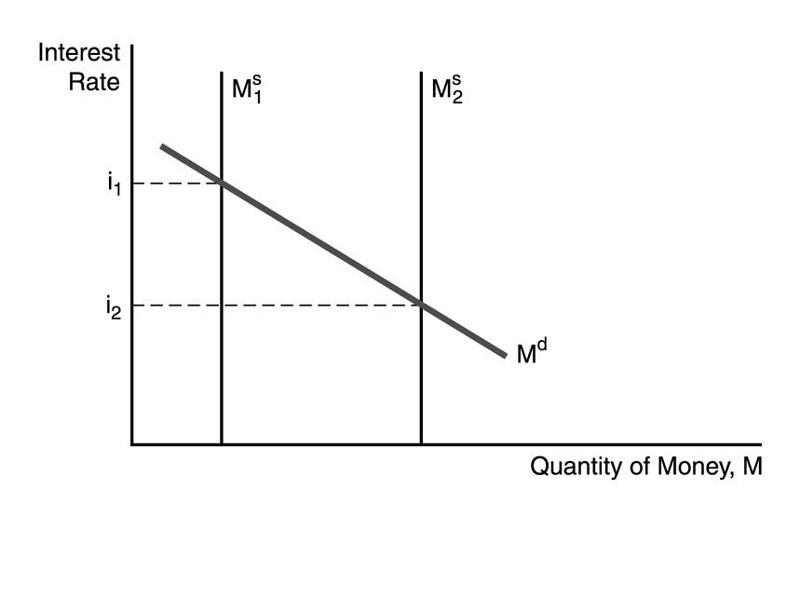In the figure above, the factor responsible for the decline in the interest rate is

1. A) a decline the price level.
2. B) a decline in income.
3. C) an increase in the money supply.
4. D) a decline in the expected inflation rate.

124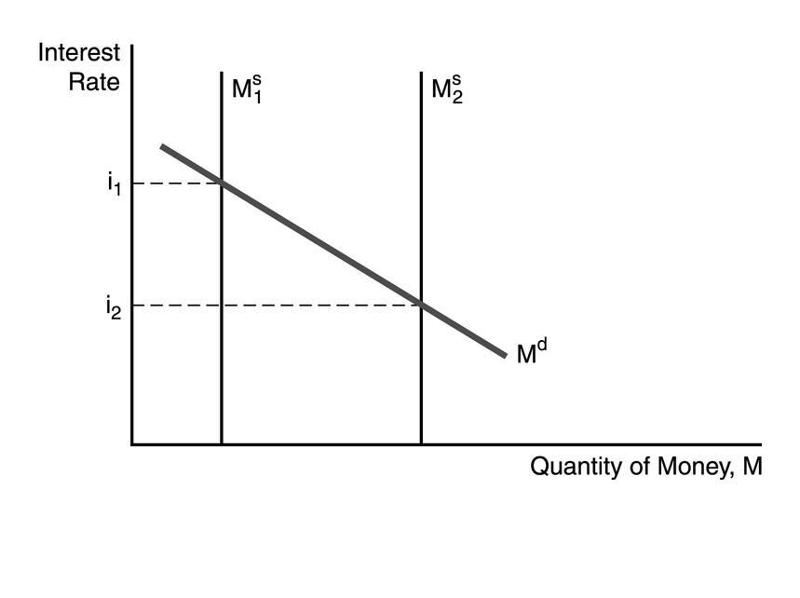In the figure above, the decrease in the interest rate from i1 to i2 can be explained by

1. A) a decrease in money growth.
2. B) an increase in money growth.
3. C) a decline in the expected price level.
4. D) an increase in income.

125

Using the liquidity preference framework, what will happen to interest rates if the Fed increases the money supply?

Answer: The Fed's actions shift the money supply curve to the right. The new equilibrium interest rate will be lower than it was previously.

126

Using the liquidity preference framework, show what happens to interest rates during a business cycle recession.

Answer: During a business cycle recession, income will fall. This causes the money demand curve to shift to the left. The resulting equilibrium will be at a lower interest rate.

127

Milton Friedman called the response of lower interest rates resulting from an increase in the money supply the ________ effect.

1. A) liquidity
2. B) price level
3. C) expected-inflation
4. D) income

128

Of the four effects on interest rates from an increase in the money supply, the initial effect is, generally, the

1. A) income effect.
2. B) liquidity effect.
3. C) price level effect.
4. D) expected inflation effect.

129

In the liquidity preference framework, a one-time increase in the money supply results in a price level effect. The maximum impact of the price level effect on interest rates occurs

1. A) at the moment the price level hits its peak (stops rising) because both the price level and expected inflation effects are at work.
2. B) immediately after the price level begins to rise, because both the price level and expected inflation effects are at work.
3. C) at the moment the expected inflation rate hits its peak.
4. D) at the moment the inflation rate hits it peak.

130

Of the four effects on interest rates from an increase in the money supply, the one that works in the opposite direction of the other three is the

1. A) liquidity effect.
2. B) income effect.
3. C) price level effect.
4. D) expected inflation effect.

131

It is possible that when the money supply rises, interest rates may ________ if the ________ effect is more than offset by changes in income, the price level, and expected inflation.

1. A) fall; liquidity
2. B) fall; risk
3. C) rise; liquidity
4. D) rise; risk

132

When the growth rate of the money supply increases, interest rates end up being permanently lower if

1. A) the liquidity effect is larger than the other effects.
2. B) there is fast adjustment of expected inflation.
3. C) there is slow adjustment of expected inflation.
4. D) the expected inflation effect is larger than the liquidity effect.

133

When the growth rate of the money supply is increased, interest rates will fall immediately if the liquidity effect is ________ than the other money supply effects and there is ________ adjustment of expected inflation.

1. A) larger; fast
2. B) larger; slow
3. C) smaller; slow
4. D) smaller; fast

134

If the Fed wants to permanently lower interest rates, then it should raise the rate of money growth if

1. A) there is fast adjustment of expected inflation.
2. B) there is slow adjustment of expected inflation.
3. C) the liquidity effect is smaller than the expected inflation effect.
4. D) the liquidity effect is larger than the other effects.

135

If the liquidity effect is smaller than the other effects, and the adjustment to expected inflation is slow, then the

1. A) interest rate will fall.
2. B) interest rate will rise.
3. C) interest rate will initially fall but eventually climb above the initial level in response to an increase in money growth.
4. D) interest rate will initially rise but eventually fall below the initial level in response to an increase in money growth.

136

If the liquidity effect is smaller than the other effects, and the adjustment to expected inflation is immediate, then the

1. A) interest rate will fall.
2. B) interest rate will rise.
3. C) interest rate will fall immediately below the initial level when the money supply grows.
4. D) interest rate will rise immediately above the initial level when the money supply grows.

137In the figure above, illustrates the effect of an increased rate of money supply growth at time period 0. From the figure, one can conclude that the

1. A) liquidity effect is smaller than the expected inflation effect and interest rates adjust quickly to changes in expected inflation.
2. B) liquidity effect is larger than the expected inflation effect and interest rates adjust quickly to changes in expected inflation.
3. C) liquidity effect is larger than the expected inflation effect and interest rates adjust slowly to changes in expected inflation.
4. D) liquidity effect is smaller than the expected inflation effect and interest rates adjust slowly to changes in expected inflation.

138In the figure above, illustrates the effect of an increased rate of money supply growth at time period 0. From the figure, one can conclude that the

1. A) Fisher effect is dominated by the liquidity effect and interest rates adjust slowly to changes in expected inflation.
2. B) liquidity effect is dominated by the Fisher effect and interest rates adjust slowly to changes in expected inflation.
3. C) liquidity effect is dominated by the Fisher effect and interest rates adjust quickly to changes in expected inflation.
4. D) Fisher effect is smaller than the expected inflation effect and interest rates adjust quickly to changes in expected inflation.

139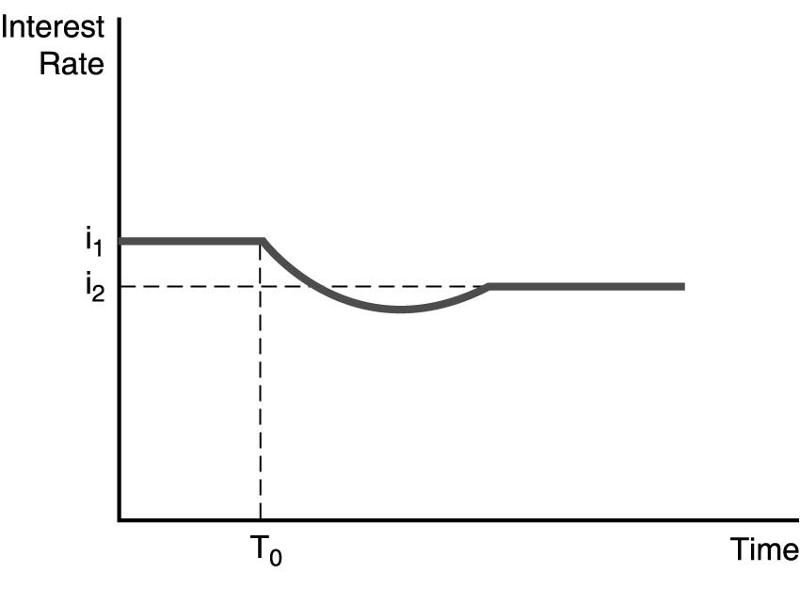The figure above illustrates the effect of an increased rate of money supply growth at time period T0. From the figure, one can conclude that the

1. A) liquidity effect is smaller than the expected inflation effect and interest rates adjust quickly to changes in expected inflation.
2. B) liquidity effect is larger than the expected inflation effect and interest rates adjust quickly to changes in expected inflation.
3. C) liquidity effect is larger than the expected inflation effect and interest rates adjust slowly to changes in expected inflation.
4. D) liquidity effect is smaller than the expected inflation effect and interest rates adjust slowly to changes in expected inflation.

140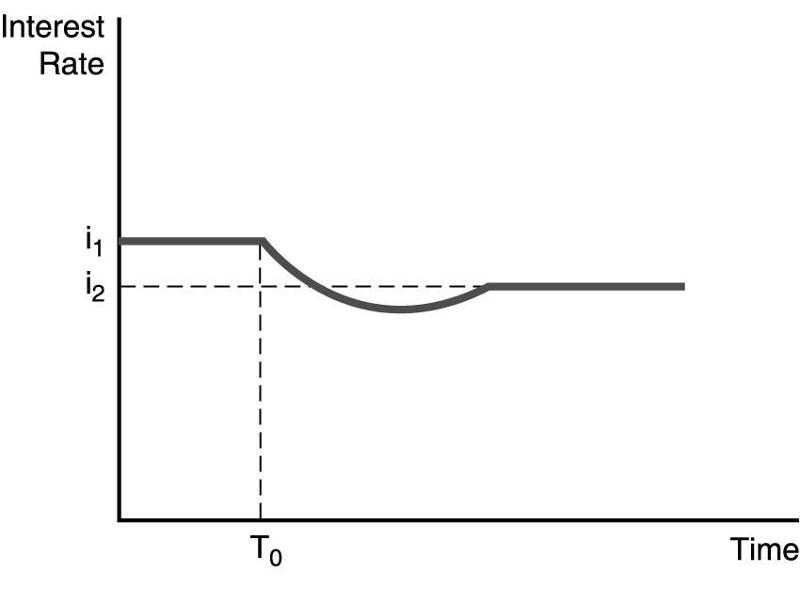The figure above illustrates the effect of an increased rate of money supply growth at time period T0. From the figure, one can conclude that the

1. A) Fisher effect is dominated by the liquidity effect and interest rates adjust slowly to changes in expected inflation.
2. B) liquidity effect is dominated by the Fisher effect and interest rates adjust slowly to changes in expected inflation.
3. C) liquidity effect is dominated by the Fisher effect and interest rates adjust quickly to changes in expected inflation.
4. D) Fisher effect is smaller than the expected inflation effect and interest rates adjust quickly to changes in expected inflation.

141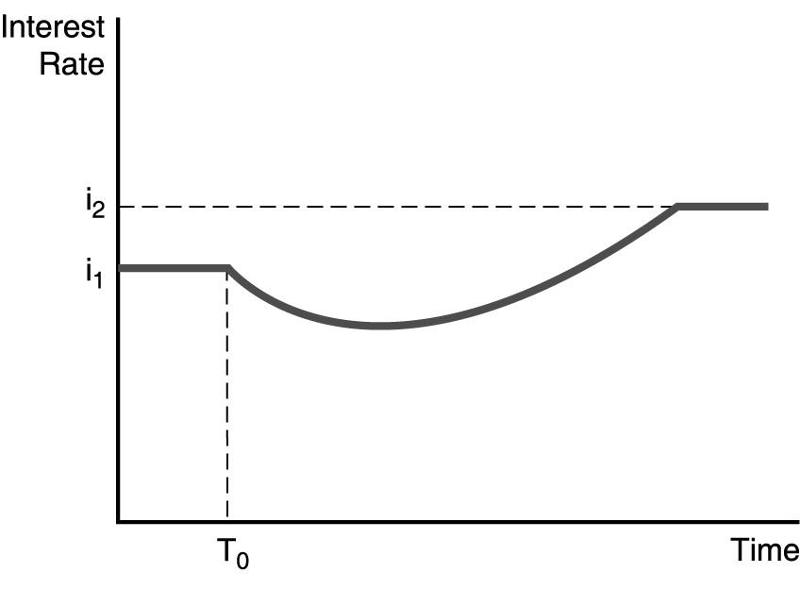The figure above illustrates the effect of an increased rate of money supply growth at time period T0. From the figure, one can conclude that the

1. A) liquidity effect is smaller than the expected inflation effect and interest rates adjust quickly to changes in expected inflation.
2. B) liquidity effect is larger than the expected inflation effect and interest rates adjust quickly to changes in expected inflation.
3. C) liquidity effect is larger than the expected inflation effect and interest rates adjust slowly to changes in expected inflation.
4. D) liquidity effect is smaller than the expected inflation effect and interest rates adjust slowly to changes in expected inflation.

142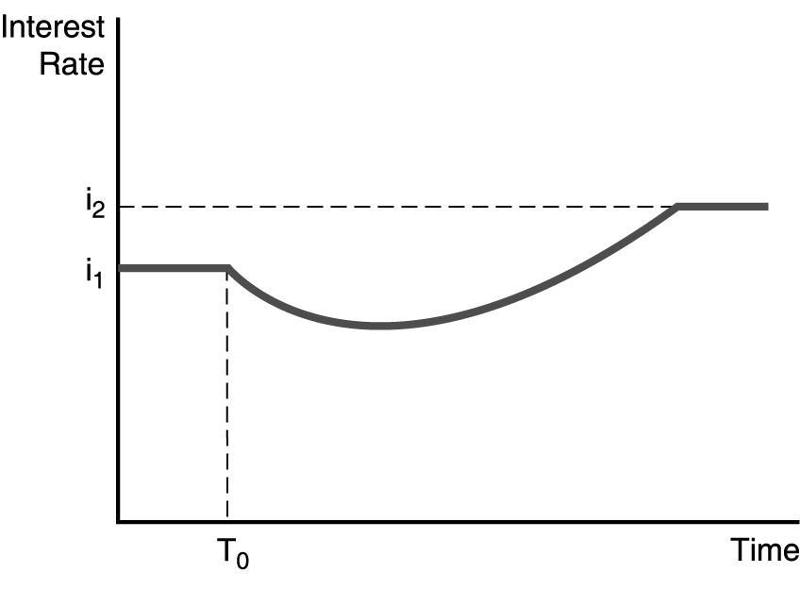The figure above illustrates the effect of an increased rate of money supply growth at time period T0. From the figure, one can conclude that the

1. A) Fisher effect is dominated by the liquidity effect and interest rates adjust slowly to changes in expected inflation.
2. B) liquidity effect is dominated by the Fisher effect and interest rates adjust slowly to changes in expected inflation.
3. C) liquidity effect is dominated by the Fisher effect and interest rates adjust quickly to changes in expected inflation.
4. D) Fisher effect is smaller than the expected inflation effect and interest rates adjust quickly to changes in expected inflation.

143

Interest rates increased continuously during the 1970s. The most likely explanation is

1. A) banking failures that reduced the money supply.
2. B) a rise in the level of income.
3. C) the repeated bouts of recession and expansion.
4. D) increasing expected rates of inflation.

144

The riskiness of an asset is measured by

1. A) the magnitude of its return.
2. B) the absolute value of any change in the asset's price.
3. C) the standard deviation of its return.
4. D) risk is impossible to measure.

145

Holding many risky assets and thus reducing the overall risk an investor faces is called

1. A) diversification.
2. B) foolishness.
3. C) risk acceptance.
4. D) capitalization.

146

The ________ the returns on two securities move together, the ________ benefit there is from diversification.

1. A) less; more
2. B) less; less
3. C) more; more
4. D) more; greater

147

A higher ________ means that an asset's return is more sensitive to changes in the value of the market portfolio.

1. A) alpha
2. B) beta
3. C) CAPM
4. D) APT

148

The riskiness of an asset that is unique to the particular asset is

1. A) systematic risk.
2. B) portfolio risk.
3. C) investment risk.
4. D) nonsystematic risk.

149

The risk of a well-diversified portfolio depends only on the ________ risk of the assets in the portfolio.

1. A) systematic
2. B) nonsystematic
3. C) portfolio
4. D) investment

150

Both the CAPM and APT suggest that an asset should be priced so that it has a higher expected return

1. A) when it has a greater systematic risk.
2. B) when it has a greater risk in isolation.
3. C) when it has a lower systematic risk.
4. D) when it has a lower systematic risk and a lower risk in isolation.

151

In contrast to the CAPM, the APT assumes that there can be several sources of ________ that cannot be eliminated through diversification.

1. A) nonsystematic risk
2. B) systematic risk
3. C) credit risk
4. D) arbitrary risk

152

When stock prices become more volatile, the ________ curve for gold shifts right and gold prices ________, everything else held constant.

1. A) demand; increase
2. B) demand; decrease
3. C) supply; increase
4. D) supply; decrease

153

A return to the gold standard, that is, using gold for money will ________ the ________ for gold, ________ its price, everything else held constant.

1. A) increase; demand; increasing
2. B) decrease; demand; decreasing
3. C) increase; supply; increasing
4. D) decrease; supply; increasing

154

When gold prices become more volatile, the ________ curve for gold shifts to the ________; ________ the price of gold.

1. A) supply; right; increasing
2. B) supply; left; increasing
3. C) demand; right; decreasing
4. D) demand; left; decreasing

155

Discovery of new gold in Alaska will ________ the ________ of gold, ________ its price, everything else held constant.

1. A) increase; demand; increasing
2. B) decrease; demand; decreasing
3. C) decrease; supply; increasing
4. D) increase; supply; decreasing

156

An increase in the expected inflation rate will ________ the ________ for gold, ________ its price, everything else held constant.

1. A) increase; demand; increasing
2. B) decrease; demand; decreasing
3. C) increase; supply; increasing
4. D) decrease; supply; increasing

157

The price of gold should be ________ to the expected inflation rate.

1. A) positively related
2. B) negatively related
3. C) inversely related
4. D) unrelated

158

In the loanable funds framework, the ________ curve of bonds is equivalent to the ________ curve of loanable funds.

1. A) demand; demand
2. B) demand; supply
3. C) supply; supply
4. D) supply; equilibrium